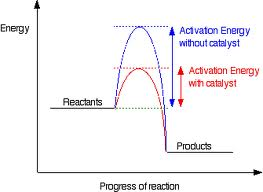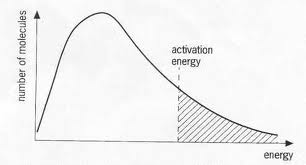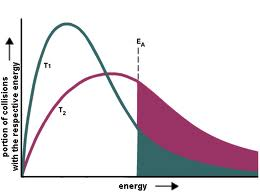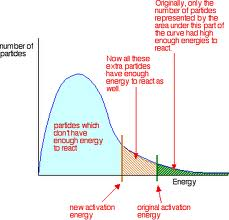# F322: Enthalpy

?

## Enthalpy

-Enthalpy is the heat content that is stored in a chemical system.
-You determine it by measuring the energy absorbed or released into the surroundings.
-The law of conservation of energy states that energy cannot be lost, only transferred from one place to another; meaning that heat loss in a chemical system = heat gain to surroundings and vice versa

-Exothermic refers to a reaction in which the enthalpy of the products is smaller than the enthalpy of the reactants, resulting in heat loss. (-ve)
.The enthalpy of products is smaller than the reactants.
.There is a heat loss from the chemical system to surroundings.
.H has a negative sign due to heat loss.

-Endothermic refers to a reaction in which the enthalpy of the products is greater than the enthalpy of the reactants, resulting in heat taken in from surroundings. (+ve)
.The enthalpy of products is greater than the reactants.
.There is a heat gain from the chemical system from the surroundings.
.H has a positive sign due to heat gain.

1 of 21

## Exothermic/Endothermic Reactions

Exothermic Reactions
-Oxidation of fuels by combustion is the most exploited exothermic reaction.
.The products have less enthalpy than the reactants, excess heat is released.
.E.g. CH4 + 2O2 -> CO2 + 2H2O           Enthalpy change = -890 kJ mol-1
-Respiration is probably the most important exothermic reaction.
.Sugars are oxidised to carbon dioxide and water.
.C6H1206 + 6O2 -> 6CO2 + 6H2           Enthalphy change = -2801 kJ mol-1

Endothermic Reactions
-Thermal decomposition of limestone is important in the fact it makes CaO, this 'lime' is crucial in making cerment and acid soil.
.CaCo3 -> CaO + CO2          Enthalpy change = +178 kJ mol-1
-Photosynthesis is probably the most important endothermic reaction.
.Sugars are made from carbon dioxide and water, light from the sun provides the energy to undertake photosynthesis.
.6CO2 + 2H2O -> C6H12O6 + 6O2          Enthalpy change = +2801 kJ mol-1

2 of 21

## Enthalpy Diagrams (Exothermic)

Exothermic-H products < H reactants.
-Activation energy is needed to break the first bond and start the reaction.
-Graph goes down

3 of 21

## Enthalpy Diagrams (Endothermic)

Endothermic-H products > H reactants.
-Graph goes up.

4 of 21

## Standard Enthalpy Changes

-Standard conditions are a pressure of 1 atmosphere, a stated temperature (i.e. 298K/25 degrees) and a concentration of 1 mol dm-3.
-Standard state is the physical state of a substance under standard conditions.

Standard enthalpy change of reaction is the enthalpy change that accompanies a reaction in the molar quantaties expressed in a chemical equation under standard conditions, for this we need an equation that gives the amount of mol of reactants/products and an enthalpy change in kJ mol-1.
H2    +    1/2O2 ->     H2O
1mol       1/2mol        1 mol          Hr = -286 kJ mol-1

Standard enthalphy change of combustion is the enthalpy change that takes place when one mole of a substance reacts completely with oxygen under standard conditions. For ethane:
C2H6 + 3.5O2 -> 2CO2 + H2O           Hc = -1560 kJ mol-1

Standard enthalphy change of a formation is the enthalphy change that takes place when one mole of a compound is formed from its constituent elements under standard conditions.
For water: H2 + 1/2O2 -> H2O           Hf = -286 kJ mol-1

[LEARN DEFINITIONS ABOVE]

5 of 21

## Determination Of Enthalpy Changes

-To determine heat exchange during a reaction, you need to know:
.m - the mass of the surroundings heated.
.c (specific heat capacity) - of the surroundings; usually 4.18.
.T - the temperature change of the surroundings.
-The surroundings are usually where the thermometer is (i.e. a beaker of water).

-For simple solutions it is possible to use a calorimeter (most simply a polystyrene cup), the insulation traps the energy and in an exothermic reaction the heat is trapped and temperature goes up, for endothermic heat is taken in from the surroundings and the temperature goes down.

6 of 21

## Enthalpy Change Of Combustion

-Combustion is the formation of oxides, and Hc is the enthalpy change that accompanies the reaction of complete combustion of one mole of a substance.
-CH4 + 2O2 -> CO2 + 2H2O          Hc = -890 kJ mol-1
-H2 + 1/2O2 -> H2O          Hc = -286 kJ mol-1

-To expermentally determine the Hc of a liquid fuel, all you need to know:
.Burn a known mass of a substance in air;
.To heat a known mass of water;
.and measure the temperature change in the water.

-In a controlled experiment the Hc value is -2061, however in a student test it's measured as slightly less at -1876; this could be because:
.There may have been incomplete combustion.
.There may have been heat loss to the surroundings; requires insulation/lid.

7 of 21

## Bond Enthalpies

-Bond enthalpy is the enthalpy change that takes place when breaking by homolytic fission 1 mol of a given bond in the molecules of a gaseous species.
-When bonds break = endothermic; when bonds are made = exothermic.
B = End                                              M = Ex(ic)o

-A bond making follows a bond breaking, breaking is endothermic and requires energy.
-Energy is then released as new bonds are formed, being exothermic.
-Furthermore in an exothermic reaction, the bonds that are formed are stronger than the bonds that are broken.
-Similarly in endothermic reactions, the bonds that are formed are weaker than those broken.

8 of 21

## Enthalpy Changes From Hc

-It's not always possible to measure enthalpy change directly due to a high activation energy, a slow reaction rate or multiple reactions; so chemists use Hess' law.
-Hess' law states that if a reaction can take place by more than one route and the initial and final conditions are the same; the total enthalpy change is the same for both.
-E.g. Route1 Reactants -> Products           Route2 Reactants -> Intermediate -> Products.
Route 1 = A, Route 2 = B+C THUS A=B+C9 of 21

## Enthalpy Changes From Hf

-This also applies to enthalpy changes of formation, as such:
.Route 1 = B+A            Route 2 = C          THUS C=A+B10 of 21

## Rates Of Reaction

-Rate of Reaction = change in concentration/time
mol dm-3 s-1                 mol dm-3             s

-Rate of reaction can be altered by:
.Temperature
.Pressure
.Concentration
.Surface Area
.Catalyst

-When molecules collide they must hit with sufficient energy and the correct orientation.-
-Collision theory states that with an increase of concentration gives more molecules in the same volume, thus collisions will be more frequent - and rate also increase.
-Similarly with an increase in pressure there will be the same molecules in a smaller volume, so more collisions will take place and again a greater rate of reaction.

11 of 21

## Catalysts

-A catalyst increases the rate of chemical reaction without being used up in the process, it can form an intermediate; and can later be regenerated.12 of 21

## Economic Importance Of Catalysts

-Used extensively in industry, and the production of polyethene and nylon; and also the Haber process for the production of ammonia. (N2 + 3H2 <-> 2NH3)
-Heterogenous catalysis is where the catalyst has a different physical state to the reactants, similarly homogenous is where they are the same.
-Enzymes are often used as biological catalysts, in things such as fermentation.

-In industry they:
.Lower temperatures required for a reaction to take place; reducing costs.
.Allow for the production of primarily pure products; removing need for seperation.
.Enzymes are biodigradable and usually not poisonous/harmful.

13 of 21

## Boltzmann Distribution

-The Boltzmann distribution is the distribution of energies of molecules at a particular temperature; often shown on a graph.
-Important features:
.The area under the curve is equal to the total number of molecules, and is unchanging.
.There are no molecules with zero energy in the system.
.There is no maximum energy for a molecule, never touching the energy axis.
.Only the molecules with energy greater than Ea can react.14 of 21

## Boltzmann Distribution (Effect Of Temperature)

-Effect of temperature-Rate increases as:
.More collisions take place take place, and there is more kinetic energy.
.A higher proportion of molecules have enough energy to react, so more succesful collisions.

15 of 21

## Boltzmann Distribution (Effect Of Catalyst)

-The activation energy is reduced:-Ec = a greater proportion of molecules exceed the lower activation energy, so reaction rate increases.

16 of 21

## Chemical Equilibrium

-Reversible reactions are indicated by a double headed arrow, they can reach equilibrium.
-Dynamic equilibrium is the equilibrium that exists in a closed system when the rate of the forward reaction is equal to the rate of the reverse reaction. (LEARN)
-A chemical system is in dynamic equilibrium when:
.The concentrations of the reactants/products remain the same.
.The rate of the forward reaction is the same as the reverse.

-le Chatelier's principle states that when a system in dynamic equibrilium is subjected to a change, the position will shift to minimise that change. (LEARN)
-Position can be affected by:
.Concentrations of the reactants/products.
.Pressure (in gases).
.Temperature.

17 of 21

## Effect Of Concentration On Chemical Equilibrium

-Increasing the concentration of a reactant causes the equilibrium to move in the direction that decreases this increased reactant concentration.
.The system opposes the change by decreasing the concentration of the reactant by removing it.
.The position of equilibrium moves to the right-hand side, forming more products.

-Increasing the concentration of a product causes the equilibrium to move in the direction that deceases this increased product concentration.
.The system opposes the change by decreasing the concentration of the product by removing it.
.The position of the equilibrium moves to the left-hand side, forming more reactants.

18 of 21

## Effect Of Pressure On Chemical Equilibrium

-Changing total pressure only changes the position if both reactant and products are gases.
N2 + 3H2           <->            2NH3
4 mol                               2 mol
higher pressure              lower pressure

-In total there are 4 moles of gas on the left, and two on the right; so the left hand side has greater pressure.

-Increasing the total pressure of the system causes the system to move to the side with fewer gas molecules, as this will decrease pressure - in this it will move to the right.
-Decreasing the total pressure of the system causes the system to move to the side with more gas molecules, to increase the pressure - in this it will move to the left.

19 of 21

## Effect Of Temperature On Chemical Equilibrium

-Increasing temperature of the system causes the position of equilibrium to move in the direction that decreases the temperature.
.The system opposes the change by taking in heat and the position moves to the left.
.The position moves in the endothermic direction (+ve).

-Decreasing temperature of the system causes the position of equilibrium to move in the direction that increases the temperature.
.The system opposes the change by releasing heat and the position moves to the right.
.The position moves in the exothermic direction (-ve).

-Additionally, a catalyst does not alter position of equilibrium; it speeds up the rate of both reactions equally - and increases the rate at which equilibrium is established.

20 of 21

## Equilibrium Of Industry

-Examples:
.The preperation of ammonia from N and H in the Haber process.
.The conversion of sulfur dioxide into sulfur trioxide by the Contact process.
Haber process =    N2 + 3H2 <-> 2NH3             H= -93 kJ mol-1
4 moles                              2 moles

-This reaction favours (as the production of ammonia is the forward reaction):
.Using high pressure. (4->2 moles)
.Using low temperatures. (exothermic)
However low temperatures provide a slower reaction, and high pressure is expensive.

-Compromise:
.Temperature of 400-500 degrees C.
.A high pressure that is safe - 200 atmospheres.
.An iron catalyst to speed it up.

21 of 21